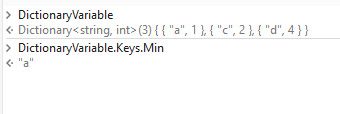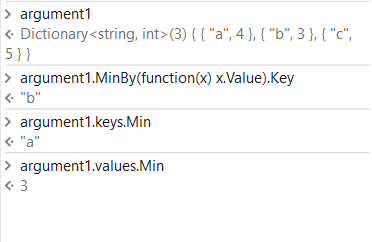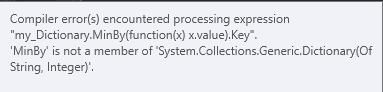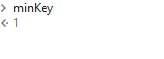# Get the key from dictionary where value is min

Hello, first post here.

Need some help with getting the key-value from a dictionary where the value is min.
Dictionary is str and int and looks like this:
{“a”, 2 : “b”, 1: “c”, 5}

How can I get “b” from the dict by finding that 1 is the min value?
or if the dictionary look like this:
{“a”, 2 : “b”, 5: “c”, 1 : “d”, 1}
How do I get the first key where on min vaule?
In this case “c”.

I come from python, so I might be thinking a bit much like how to do it there.
ex: min_key = min(my_dict, key=my_dict.get)

I can’t find a good way to do this, help please

BR S

Hi @Sven_son ,

Try

DictionaryVariable.Keys.Min1 Like

@Sven_son

I guess you want the key where the value is minimum…

Then use as below

`Dict.MinBy(function(x) x.Value).Key` - gets the key related to minimum value
`Dict.Keys.Min` - gets minimum key from the keys
`Dict.values.Min` - gets the minimum value from the valuesHope this helps

Cheers

2 Likes

Hi @Sven_son ,

DictionaryVariable.MinBy(function(x) x.value).Key

To get the key with respect to the minimum value, the previous expression was for only the minimum key.

1 Like

I got an error using “my_Dictionary.MinBy(function(x) x.value).Key”Hi @Sven_son ,
Can you display the values of dictionary by debugging?

You can use the following too:

DictionaryVariable.OrderBy(function(x) x.Value).First.key

1 Like

“DictionaryVariable.OrderBy(function(x) x.Value).First.key”

That seems to work better. Im gonna do some more testing. And if it works as good as i looks now I’ll set this to solution